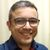# QlikView App Dev

Discussion Board for collaboration related to QlikView App Development.

Announcements
Join this live chat April 6, 10AM EST - QlikView to Qlik Sense REGISTER
cancel
Showing results for
Search instead for
Did you mean:Contributor II

## sum where condition

Hello,

I have a 3 pivot tables  with dimention the store and 3 expressions

 people               counter transactions CR store1        100 15 15% store 2          30 7 23% store 3            0 5 0%

The CR expression is column(2)/column(1)  and the other two expressions are sum(people counter) and sum(transactions)

The first pivot is for the day the 2nd for the month and the 3rd for the year.

For some days the counter of the people doesnt work properly and gives 0.When I want to calculate the total CR for the year or the month

I want to not to sum the days where the CR was 0 .

Can somone give the set analysis where the expression will  the sum of transactions WHERE THE PEOPLE COUNTER WAS NOT 0?

Thank you in advance

4 RepliesMVP

May be like this

Sum({<Store = {"=Sum([people counter]) <> 0"}>}transactions)Luminary Alumni

When applicable please mark the appropriate replies as CORRECT. This will help community members and Qlik Employees know which discussions have already been addressed and have a possible known solution. Please mark threads as HELPFUL if the provided solution is helpful to the problem, but does not necessarily solve the indicated problem. You can mark multiple threads as HELPFUL if you feel additional info is useful to othersContributor II
Author

Hello Sunny and thank you for your answer.

I tried your suggetion ,it didn't work but I changed it to

Sum({<date = {"=Sum([people counter]) <> 0"}>}transactions) and it worked.

My problem is that the pivot is a little more complicated than I described in the initial post

In the month table for example for counting the transactions I have the expression

Sum({<date = {">=\$(=MonthStart(Max(trn_date))) <=\$(=Max(trn_date))"}>} transactions)

(the user selects one day and sees the sum of the transactions from the beggining of the month until the chossen day)

How can I insert the condition that the above sum(of the transactions) excludes the days where the people counter was 0.

I tried something like :

Sum({<date = {">=\$(=MonthStart(Max(trn_date))) <=\$(=Max(trn_date))"}>*<date = {"=Sum([people counter]) <> 0"}>} transactions)

but it retruns 0.

Sorry if my questions are novice of silly,but I dont have the experience to understand the mistake.MVP

May be this

Sum({<date = {"=Sum([people counter]) <> 0 and date >= MonthStart(Max(trn_date)) and date <= Max(trn_date)"}>} transactions)